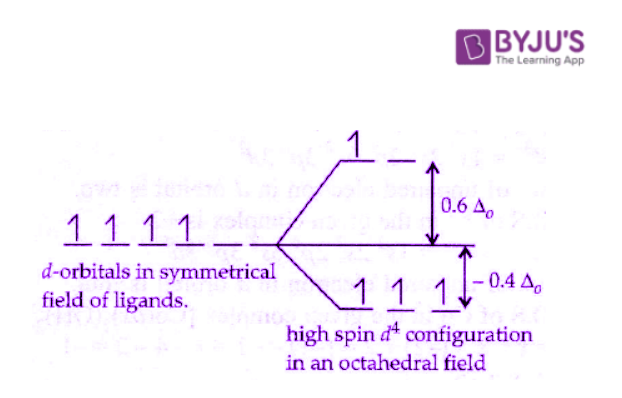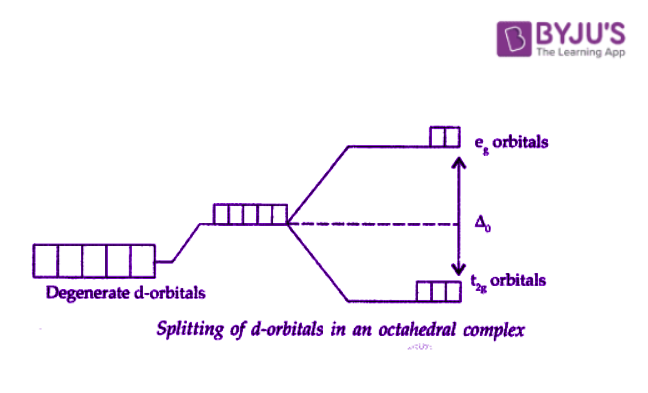Checkout JEE MAINS 2022 Question Paper Analysis : Checkout JEE MAINS 2022 Question Paper Analysis :

# Crystal Field Theory Questions

Crystal field theory was established in 1929 and treats the interaction of metal ion and ligands as a purely electrostatic phenomenon where the ligands are considered as point charges in the vicinity of the atomic orbitals of the central atom. Development and extension of crystal field theory takes into account the partly covalent nature of bonds between the ligand and metal atom mainly through the application of molecular orbital theory.

 Definition: Crystal field theory describes the net change in crystal energy resulting from the orientation of d orbitals of a transition metal cation inside a coordinating group of anions also called ligands.

## Crystal Field Theory Chemistry Questions with Solutions

Q1. Which of the following compound is paramagnetic?

a.) Hexa amine chromium (III) ion

b.) Tetraamminezinc (II) ion

c.) Tetracyanonickelate (II) ion

d.) Diammine silver (I) ion

Correct Answer– (a.) Hexa amine chromium (III) ion.

Explanation– A complex is paramagnetic due to the presence of unpaired electrons. Chromium contains 3 unpaired electrons.

Q2. Crystal field stabilization energy for high spin d4 octahedral complex is:

a.) – 1.8 Δ0

b.) – 1.6 Δ0 + P

c.) –1.2 Δ0

d.) –0.6 Δ0

Explanation–CFSE = 3 ( –0.4)Δ0 + 0.6Δ0

= –1.2Δ0 + 0.6Δ0 = –0.6Δ0

Q3. Which one of the following statements is FALSE?

a.) In an octahedral crystal field, the d electrons on a metal ion occupy the eg set of orbitals before occupying the t2g set of orbitals.

b.) Diamagnetic metal ions cannot have an odd number of electrons.

c.) Low spin complexes can be paramagnetic.

d.) In high spin octahedral complexes, Δoct is less than the electron pairing energy and is relatively very small.

Correct Answer– (a.) In an octahedral crystal field, the d electrons on a metal ion occupy the eg set of orbitals before occupying the t2g set of orbitals.

Q4. What will be CFSE for the d6 high spin complex for both tetrahedral and octahedral complexes?

a.) 0.6, 0.6

b.) 0.4, 0.4

c.) 0.4, 0.6

d.) 0.6, 0.4

Q5. According to crystal field theory-

a.) The order of increasing field strength of ligands is F > H2O > NH3.

b.) If pairing energy exceeds the magnitude of crystal field splitting, then pairing occurs.

c.) d4 to d7 coordination entities are more stable for the strong field than weak field cases.

d.) Tetrahedral complexes have sufficiently large splitting energy to force pairing, and therefore, high spin configurations are rarely observed.

Correct Answer– (c.) d4 to d7 co-ordination entities are more stable for the strong field as compared to weak field cases.

Q6. Fill in the blank.

The electronic configuration of the central atom is K4(Fe(CN)6] on crystal field theory is ___.

Answer. The electronic configuration of the central atom is K4(Fe(CN)6] on crystal field theory is t2g6eg0.

Q7. State True or False.

Strong field ligands, such as CN usually produce low spin complexes and large crystal field splitting.

Strong field ligands, such as CN usually produce low spin complexes and large crystal field splitting.

Q8. What are the limitations of crystal field theory?

Answer. The limitations of crystal field theory are as follows:

• This theory is applicable to metal ions with d-orbitals and does not explain s- and p-orbitals.
• It does not explain z bonding in the coordination compounds.
• This theory considers electrostatic attraction between central metal ion and ligands, hence considering only ionic bonds between them but does not account for covalent nature between them or coordínate bonds.
• Water (H2O) is a stronger ligand than OH, which this theory cannot explain satisfactorily.

Q9. Explain by giving a suitable example for Crystal field splitting.

Answer. The presence of ligands causes the splitting of degenerate energy levels. When a ligand approaches a transition metal ion, the degenerate d-orbitals split into two sets, one with lower energy and one with higher energy which is referred to as crystal field splitting, and the difference between the lower and higher energy sets is referred to as crystal field splitting energy (CFSE).Example: 3d5 of Mn2+

Q10. On the basis of crystal field theory, write the electronic configuration of d4 in terms of t2g and eg in an octahedral field when Δ0 < P.

Answer. The magnitude of Δ0 decides the actual configuration of d-orbitals with the help of mean pairing energy.

If P < Δ0, then pairing of electrons occurs within the same set and forms low spin complexes due to strong-field ligands. Therefore, it becomes more energetically favourable for the fourth electron to occupy a t2g orbital with configuration t2g4 eg0.

Q11. If Δ0 is the octahedral crystal field splitting energy. Then what will be the CFSE for Fe(CN)6]4?

Answer. Fe is in +2 oxidation state, and therefore the configuration will be 3d6.

Since the ligand is a strong field ligand, the excitation energy is greater than the pairing energy. Therefore, the configuration is t2g6 eg0.

CFSE= 6 × (– 0.4Δ0) = – 2.4 Δ0.

Q12. How is the crystal field splitting energy for octahedral(Δ0) and tetrahedral (Δt) complexes related?

Answer. Splitting in tetrahedral complexes is 2/3rd of the octahedral complex.

Therefore, for one ligand splitting on octahedral

$$\begin{array}{l}=\frac{\Delta _{0}}{6}\end{array}$$
, For one ligand splitting in tetrahedral is
$$\begin{array}{l}=\frac{2}{3}\left ( \frac{\Delta _{0}}{6} \right )\end{array}$$

Therefore, for four ligand, [latex\Delta t=4\times \frac{2}{3}\left ( \frac{\Delta _{0}}{6} \right )=\frac{4}{9}\Delta _{0}\end{array} \)

The relationship of crystal field splitting energy for octahedral(Δ0) and tetrahedral (Δt) complexes is-
$$\begin{array}{l}\Delta t=\frac{4}{9}\Delta _{0}\end{array}$$

Q13. How does the magnitude of Δ0 decide the actual configuration of d orbitals in a coordination entity?

Answer. If Δ0 is greater than the pairing energy, electrons get paired in lower energy d-orbitals giving a low spin complex. On the other hand, if Δ0 is, is less than the pairing energy, electrons occupy higher energy d-orbitals giving a high spin complex.

Q14. Explain the violet colour of [Ti(H2O)6]3+ complex on the basis of the crystal field theory?

Answer. [Ti(H2O)6]3+ is an octahedral complex. The oxidation state of Ti is +3 with the coordination number 6.

Its outer electronic configuration is 3d1, which means that it has one unpaired electron. This unpaired electron is excited from t2g level to eg level by absorbing yellow light and hence appears violet coloured.

Q15. Based on crystal field theory, write the electronic configuration of d4 in terms of t2g and eg in an octahedral field when Δ0 > P.

Answer. The magnitude of Δ0 decides the actual configuration of d-orbitals with the help of mean pairing energy.

If P > Δ0, then pairing of electrons does not occur, and electrons enter the higher energy orbitals and thus form high spin complexes due to weak-field ligands. Therefore, one electron enters in eg orbital and 3 electrons in t2g with configuration t2g3 eg1.

## Practise Questions on Crystal Field Theory

Q1. How many unpaired electrons are there in a strong field iron(II) octahedral complex?

a.) 0

b.) 1

c.) 2

d.) 4

Q2. Strong field ligands such as CN:

a.) usually produce high spin complexes and small crystal field splittings.

b.) usually produce low spin complexes and small crystal field splittings.

c.) usually produce low spin complexes and high crystal field splittings.

d.) usually produce high spin complexes and high crystal field splittings.

Q3. The hexaaquo manganese (II) ion contains five unpaired electrons while the hexacyano ion contains only one unpaired electron. Explain using Crystal Field Theory.

Q4. Why does Co2+ readily oxidise to Co3+ in complexes?

Q5. What is the Difference Between VBT and CFT?

Click the PDF to check the answers for Practice Questions.

## Octahedral Complexes